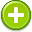calculate 中文解釋 wordnet sense Collocation Usage
Verb
/ˈkalkyəˌlāt/,Font size:calculates, 3rd person singular present; calculating, present participle; calculated, past participle; calculated, past tense;
1. Determine (the amount or number of something) mathematically
• - Japanese land value was calculated at 2.5 times that of the U.S
• - he calculated that Texas would gain four new seats in the House of Representatives
2. Determine by reasoning, experience, or common sense; reckon or judge
• - I was bright enough to calculate that she had been on vacation
3. Include as an essential element in one's plans
• - he may have calculated on maximizing pressure for policy revision
4. Intend (an action) to have a particular effect
• - his last words were calculated to wound her
5. Suppose; believe

1. make a mathematical calculation or computation
2. judge to be probable
3. account: keep an account of
5. specifically design a product, event, or activity for a certain public
6. count: have faith or confidence in; "you can count on me to help you any time"; "Look to your friends for support"; "You can bet on that!"; "Depend on your family in times of crisis"
7. A calculation is a deliberate process for transforming one or more inputs into one or more results, with variable change.
8. Calculated is the only full-length record by American punk band Heavens to Betsy. It was released on January 1, 1994 on Kill Rock Stars.
9. (Calculation (game)) Calculation (also known as Broken IntervalsMorehead A. (1977). "The Complete Book of Solitaire & Patience Games", ISBN 0-553-20621-4, pg 83) is a solitaire card game. ...
10. (mathematics) To determine the value of something or the solution to something by a mathematical process; (mathematics) To determine values or solutions by a mathematical process; To plan something, especially something morally wrong
11. (calculating) this sense?) Which has the ability to calculate; Which serves one's own interests in an unemotional, analytical manner
12. A calculated value is derived or determined by the values of other fields. This value cannot be edited directly by a user, instead the source fields must be edited and a new derived value will be calculated.